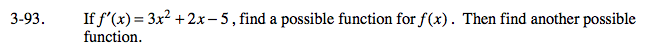### Home > CALC > Chapter Ch3 > Lesson 3.3.1 > Problem3-93

3-93.Undo the power rule.

The 3x² in f '(x) will become x³ in f(x). What will happen to the 2x and the −5?

The 2x in f '(x) will become x² in f(x).

The −5 in f '(x)will become −5x in f(x).

Giving us f(x) = x³ + x² − 5x. But there are other functions that also have the same derivative.

To find another f(x), think about the Power Rule. What happens to a constant when you differentiate?